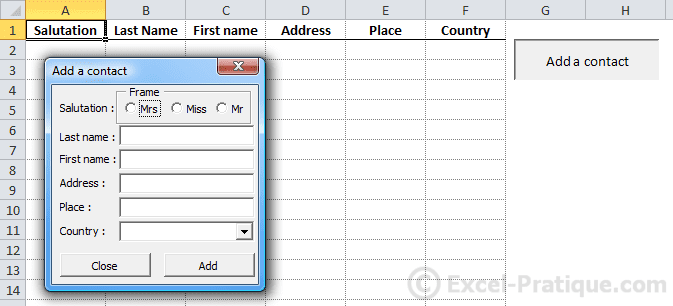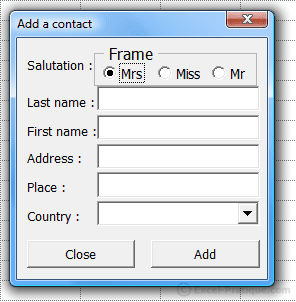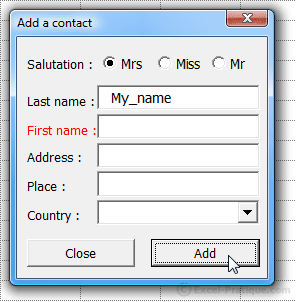# VBA Course: Controls (exercise)

Now let's try a little exercise to practice using controls ...

This is how we start the exercise:By now it should be clear that the goal here is to fill the table using the form.

A few thinks to keep in mind:

• List the countries based on the list on the second worksheet
• Verify the contents of the controls before adding a new contact
• After inserting a value, reinitialize the values of the controls without closing the form

Take a moment to work through this exercise before looking at the solution ...

.
.
.
.
.
.
.
.
.
.
.
.
.
.
.
.
.
.
.
.
.
.
.
.
.
.
.
.
.
.

## This is one way that you could solve this problem

Our first action should be to increase the Zoom property of the UserForm to 120 to make it easier to use the form:We have already covered option button tests (in the first controls page), so we are using a simpler solution here.

"Mrs" is chosen by default (Value property: True), which means that we don't have to verify that a salutation has been chosen.

## The "Close" button

`Private Sub CommandButton_Close_Click()    Unload MeEnd Sub`

## The contents of the drop-down list

`Private Sub UserForm_Initialize() 'Loading the list when the UserForm is opened    For i = 1 To 252 'List of 252 countries froim the "Country" worksheet        ComboBox_Country.AddItem Sheets("Country").Cells(i, 1)    NextEnd Sub`

## Verification of controls

One simple solution would be to display a dialog box if any of the controls is empty:

`Private Sub CommandButton_Add_Click()    If TextBox_Last_Name.Value = "" Or TextBox_First_Name.Value = "" Or TextBox_Address.Value = "" Or TextBox_Place.Value = "" Or ComboBox_Country.Value = "" Then        MsgBox "Form incomplete"    Else        'Instructions for inserting a contact here ...    End IfEnd Sub`

But to make things a bit more complicated, each control should be tested individually, and if any of them is empty, its Label text color should be changed to red:

`Private Sub CommandButton_Add_Click()    'Setting Label text color to black    Label_Last_Name.ForeColor = RGB(0, 0, 0)    Label_First_Name.ForeColor = RGB(0, 0, 0)    Label_Address.ForeColor = RGB(0, 0, 0)    Label_Place.ForeColor = RGB(0, 0, 0)    Label_Country.ForeColor = RGB(0, 0, 0)    'Content controls    If TextBox_Last_Name.Value = "" Then 'IF no "name" provided ...        Label_Last_Name.ForeColor = RGB(255, 0, 0) 'Set Label "name" color to red    ElseIf TextBox_First_Name.Value = "" Then        Label_First_Name.ForeColor = RGB(255, 0, 0)    ElseIf TextBox_Address.Value = "" Then        Label_Address.ForeColor = RGB(255, 0, 0)    ElseIf TextBox_Place.Value = "" Then        Label_Place.ForeColor = RGB(255, 0, 0)    ElseIf ComboBox_Country.Value = "" Then        Label_Country.ForeColor = RGB(255, 0, 0)    Else        'Instructions for inserting a contact here ...    End IfEnd Sub`## Inserting data

The following code should be inserted in the place indicated in the code above (see commentary):

`Dim row_number As Integer, salutation As String'Choice of SalutationFor Each salutation_button In Frame_Salutation.Controls    If salutation_button.Value Then        salutation = salutation_button.Caption 'Salutation chosen    End IfNext'row_number = row number of the last non-empty cell in the column +1row_number = Range("A65536").End(xlUp).Row + 1'Inserting values into the worksheetCells(row_number, 1) = salutationCells(row_number, 2) = TextBox_Last_Name.ValueCells(row_number, 3) = TextBox_First_Name.ValueCells(row_number, 4) = TextBox_Address.ValueCells(row_number, 5) = TextBox_Place.ValueCells(row_number, 6) = ComboBox_Country.Value'After insertion, the initial values are replacedOptionButton1.Value = TrueTextBox_Last_Name.Value = ""TextBox_First_Name.Value = ""TextBox_Address.Value = ""TextBox_Place.Value = ""ComboBox_Country.ListIndex = -1`

## Overall view

That's all, and here you have the complete code for the exercise and the downloadable Excel file:

`Private Sub CommandButton_Close_Click()    Unload MeEnd SubPrivate Sub UserForm_Initialize() 'List of 252 countries on the "Country" worksheet    For i = 1 To 252        ComboBox_Country.AddItem Sheets("Country").Cells(i, 1)    NextEnd SubPrivate Sub CommandButton_Add_Click()    'Setting Label color to black    Label_Last_Name.ForeColor = RGB(0, 0, 0)    Label_First_Name.ForeColor = RGB(0, 0, 0)    Label_Address.ForeColor = RGB(0, 0, 0)    Label_Place.ForeColor = RGB(0, 0, 0)    Label_Country.ForeColor = RGB(0, 0, 0)    'Content controls    If TextBox_Last_Name.Value = "" Then 'If no "name" provided ...        Label_Last_Name.ForeColor = RGB(255, 0, 0) 'Set Label "name" color to red    ElseIf TextBox_First_Name.Value = "" Then        Label_First_Name.ForeColor = RGB(255, 0, 0)    ElseIf TextBox_Address.Value = "" Then        Label_Address.ForeColor = RGB(255, 0, 0)    ElseIf TextBox_Place.Value = "" Then        Label_Place.ForeColor = RGB(255, 0, 0)    ElseIf ComboBox_Country.Value = "" Then        Label_Country.ForeColor = RGB(255, 0, 0)    Else        'If the form is complete, the values will be inserted onto the worksheet        Dim row_number As Integer, salutation As String                'Choice of salutation        For Each salutation_button In Frame_Salutation.Controls            If salutation_button.Value Then                salutation = salutation_button.Caption            End If        Next        'row_number = row number of the last non-empty cell in column +1        row_number = Range("A65536").End(xlUp).Row + 1        'Inserting values onto the worksheet        Cells(row_number, 1) = salutation        Cells(row_number, 2) = TextBox_Last_Name.Value        Cells(row_number, 3) = TextBox_First_Name.Value        Cells(row_number, 4) = TextBox_Address.Value        Cells(row_number, 5) = TextBox_Place.Value        Cells(row_number, 6) = ComboBox_Country.Value                'After insert, we replace the initial values        OptionButton1.Value = True        TextBox_Last_Name.Value = ""        TextBox_First_Name.Value = ""        TextBox_Address.Value = ""        TextBox_Place.Value = ""        ComboBox_Country.ListIndex = -1    End IfEnd Sub`